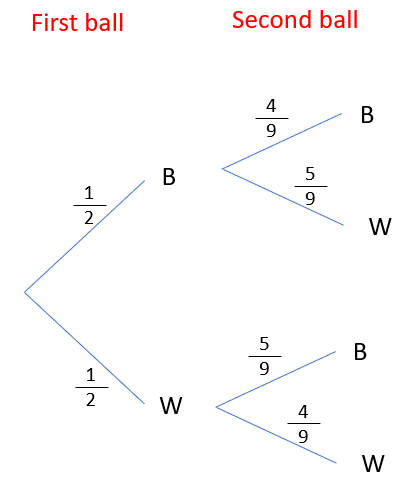• ## Accessibility

Open the accessibility toolbar to change fonts and contrast, access a dictionary, use a ruler and more

In Probability, there are two distinct types of events, conditional and independent.

Independent Events - The outcome of the first one does not affect the outcome of the second

Conditional Events - The outcome of the first event does affect the outcome of the seond.

I'm going to assume that you are happy with drawing tree diagrams. If you are unclear, I recommend completing the tree diagram worksheet first.

Example: I have a ball that contains 5 black balls and five white balls. I pull one out, don't replace it and then pull another one out.

The key thing to note here is the question says 'don't replace'. This means that we are dealing with conditional probability

Let's start by looking what could happen the first time we pull out a ballWe can get either black or white, there's 5 of each so the probabilities are both 1/2

Let's now look at the second branches. If I pulled out a black first time, I would now have 4 black and 5 white balls.

This is going to change the probabilitiesI can now do the same for white first. If I pulled out a white first, I have 5 black balls and 4 white balls.From here, I complete this the same way I would a normal probability tree.You could if you wanted to, cancel these fractions down now, I would however recommend leaving them. In a moment we might have to add them together and if they all have the same denominator it'll be easier.

We can always cancel down at the end.

How do we use this?

Tree diagrams are all about finding probabilities for combined events. Lets look at an example.

What is the probability that I pull out at least one black.

There are three ways this can happen. BB, BW and WB

All we have to do is find the ones on the tree that show this (They're the top 3) and add them together.

4/18 + 5/18 + 5/18 = 14/18 = 7/9

10 questions